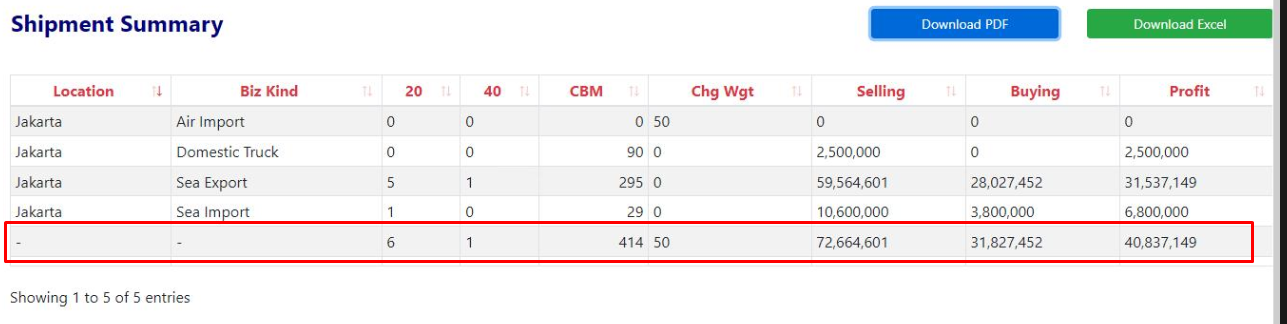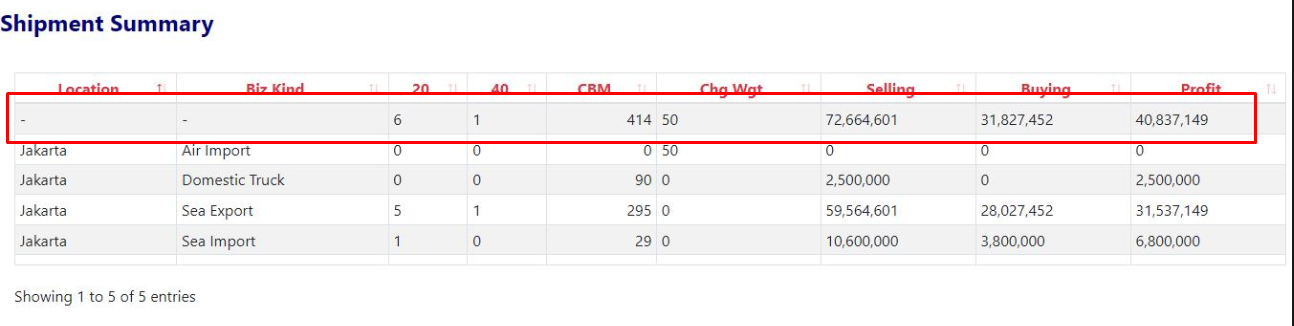# KoolReport's Forum

Official Support Area, Q&As, Discussions, Suggestions and Bug reports.
Forum's Guidelines

# How to use pipe process to create footer #2456

saiful opened this topic on on Nov 23 - 2 comments

saiful commented on Nov 23

hi, im using map process to create total row for some column like this:but it can moved to top like this:i think it was not set as footer, instead a new row.

here is my code:

``````->pipe(new \koolreport\processes\Map(array(
"{value}"=>function(\$row, \$meta, \$index, \$mapState)
{
\$totalRow = isset(\$mapState['TotalRow'])?\$mapState['TotalRow']:[]; //change 'Total" to "TotalRow" for clearer meaning
\$totalColumnNames = ["20", "40", "CBM", "Chg Wgt", "Selling", "Buying", "Profit"]; // choose which columns to do total
foreach (\$row as \$column => \$value) { // loop through the row to do total for chosen columns
if (in_array(\$column, \$totalColumnNames)) {
if(!isset(\$totalRow[\$column]))
{
\$totalRow[\$column] = 0;
}
\$totalRow[\$column] += 1 * \$value; // or 1 * \$row[\$column]
}
}

\$mapState['TotalRow'] = \$totalRow;
return ['{rows}' => \$row, '{state}' => \$mapState];
},
"{end}" => function(\$count, \$mapState)
{
\$total = \$mapState['TotalRow'];
return [\$total];
}
)))
``````

how to move the mapped data to footer?

Sebastian Morales commented on Nov 23

The total row is actually at the end of your datastore but it seems you used DataTables with ordering, which ordered the first column by default. There are two solutions:

1 . Disable ordering:

``````DataTables::create(array(
...
"options" => array(
"ordering" => false
)
));
``````

2 . Use DataTables' footer, which can be used with ordering any column:

``````DataTables::create(array(
...
"showFooter" => true,
"columns" => array(
...
"CBM" => array(
...
"footer" => "sum",
"footerText" => "@value",
),
)
));
``````
saiful commented on Nov 23

thanks, solution 2 is suitable for my project.